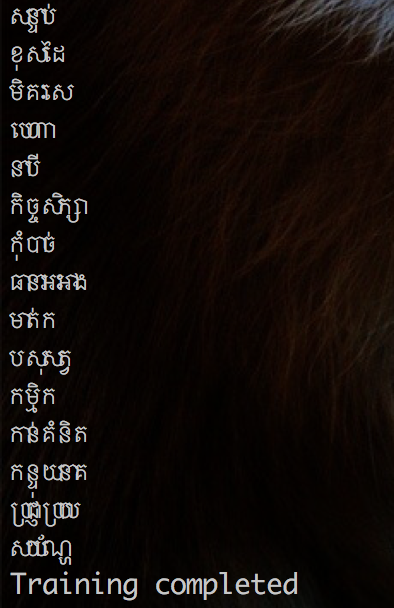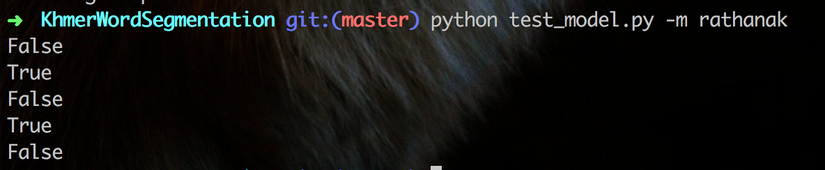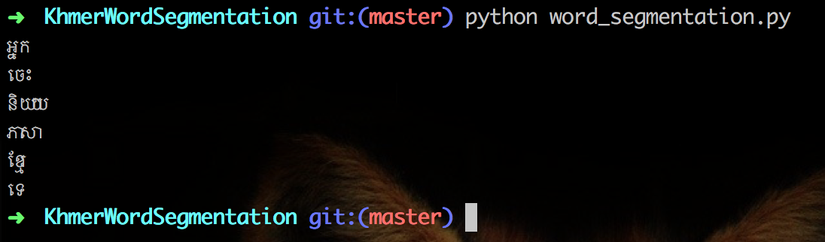Python

## NLP: Khmer Word SegmentationRathanak
https://viblo.asia/p/nlp-khmer-word-segmentation-YWOZrgNNlQ0

### Introduction

In my previous article, I talk about "Text Tokenization" on English language where we can use existed library to do the job with ease. Unlike other languages, Khmer Word Segmentation is way more complex. Because the Khmer language does not have any standard rule on how we are using space to separate between each word(space are used for easier reading). Moreover, Khmer word can have different meaning with the order of words when it will form. Khmer word could also be a join of two or more Khmer words together. Because of uncertain rule of spacing and the complicated structure above, which it is hard to segment Khmer Word.

In this article, I am going to a simple yet powerful data struture `trie` to apply on Khmer word. As we all know, we need to fit our `trie` data structure with the array of words. Then we can use the model to find/split words from given sentences which we can apply "Text Tokenization" on. So we need to find a list of Khmer words. Luckily, I found a repo which contain list of Khmer words. However, we need to write few line of code to prepare those list for our `trie` model.

### Coding

Let's start coding.

First we convert `tsv` format to `txt` format where later we can use it to fit our `trie` model. As you are notice, we need to inform and encoding our Khmer string to `utf-8`.

``````#parse_tsv_word.py
#!/usr/bin/python
# -*- coding: utf-8 -*-
# This file use to get Khmer word from tsv and save in other file

import pandas as pd
import numpy as np
from codecs import open
from argparse import ArgumentParser

parser = ArgumentParser()
parser.add_argument("-f", "--file", type=str, default="",
help="File input name", required=True)
parser.add_argument("-o", "--out", type=str, default="",
help="File output name", required=True)

args = vars(parser.parse_args())
file_path = args["file"]
output_file_path = args["out"]

words_list = pd.read_csv(file_path, delimiter="\t",
with open(output_file_path, "w", encoding="utf-8") as f:
for word in words_list[words_list.columns]:
f.write(word + "\n")
``````

Let's run the command to read `tsv` file and save it as `txt`.

``````python parse_tsv_word.py -f data/villages.tsv -o data/villages.txt
``````

Here we read the `txt` file where we prepared above to train our `trie` model(Let's assume you already have already built `trie` class).

``````#train_model.py
#!/usr/bin/python
# -*- coding: utf-8 -*-

import trie
from codecs import open, decode

model = trie.Trie()

input_file_path = "data/sea.txt"
with open(input_file_path, "r") as f:
print("Training start")
for word in words:
if not bool(word.strip()):
continue

print(word)
model.insertWord(word)

model.save_to_pickle("train_data")
print("Training completed")
``````

Then run the code to train and store in `pkl` file for later use.

``````python train_model.py
``````Next, we test our trained model and some of `trie` methods.

``````#test_model.py
#!/usr/bin/python
# -*- coding: utf-8 -*-

import trie
from codecs import open, decode
model = trie.Trie()

print(model.searchWord('')) # should False
print(model.searchWord('គ្រុយ')) # should be True
print(model.searchWord('គ្រុ')) # should be False
print(model.searchWordPrefix('គ្រុ')) # should be True
print(model.searchWordPrefix('គ្រុយ')) # should be False
``````

Let's run the code:

``````python test_model.py
``````Finally, it's time to use our model to break our very first and simple sentences:

``````#word_segmentation.py
#!/usr/bin/python
# -*- coding: utf-8 -*-

import trie
from codecs import open, decode
model = trie.Trie()

kh_str = "អ្នកចេះនិយាយភាសាខ្មែរទេ?"

words = []
word = ''
for ch in kh_str:
word += ch

if model.searchWord(word.strip()):
words.append(word)
print(word.strip())
word = ''
``````

Then run it

``````python word_segmentation.py
``````It works Awesome.

### Next Step

We have collected Khmer words from many public data sources. We then prepare those words and train them in our `Trie` model. However, the text data from those public data do not container all Khmer word. So next step, we are going to fit code with the given sentences of known and unknown words where we can store those unknow words and use them to improve our model.

Awesome.#### Bài viết liên quan